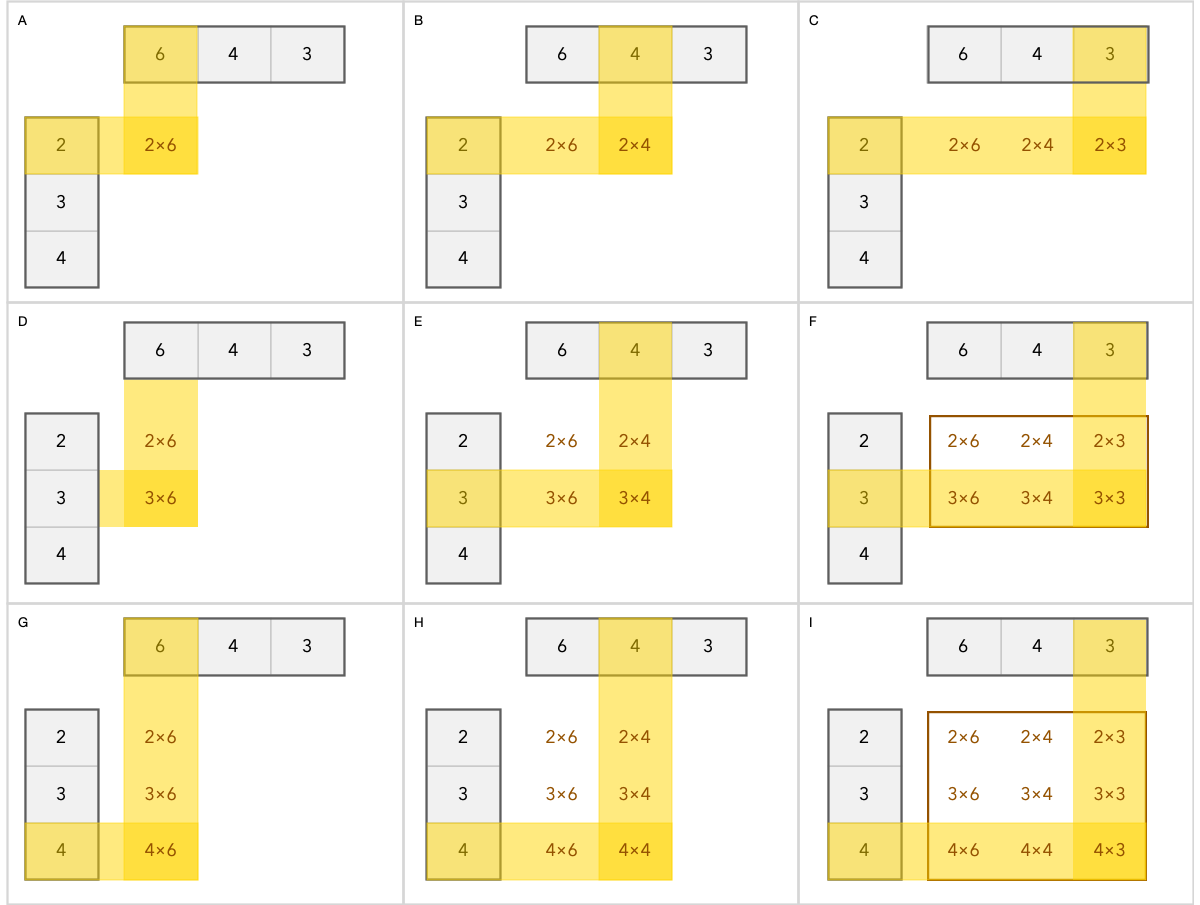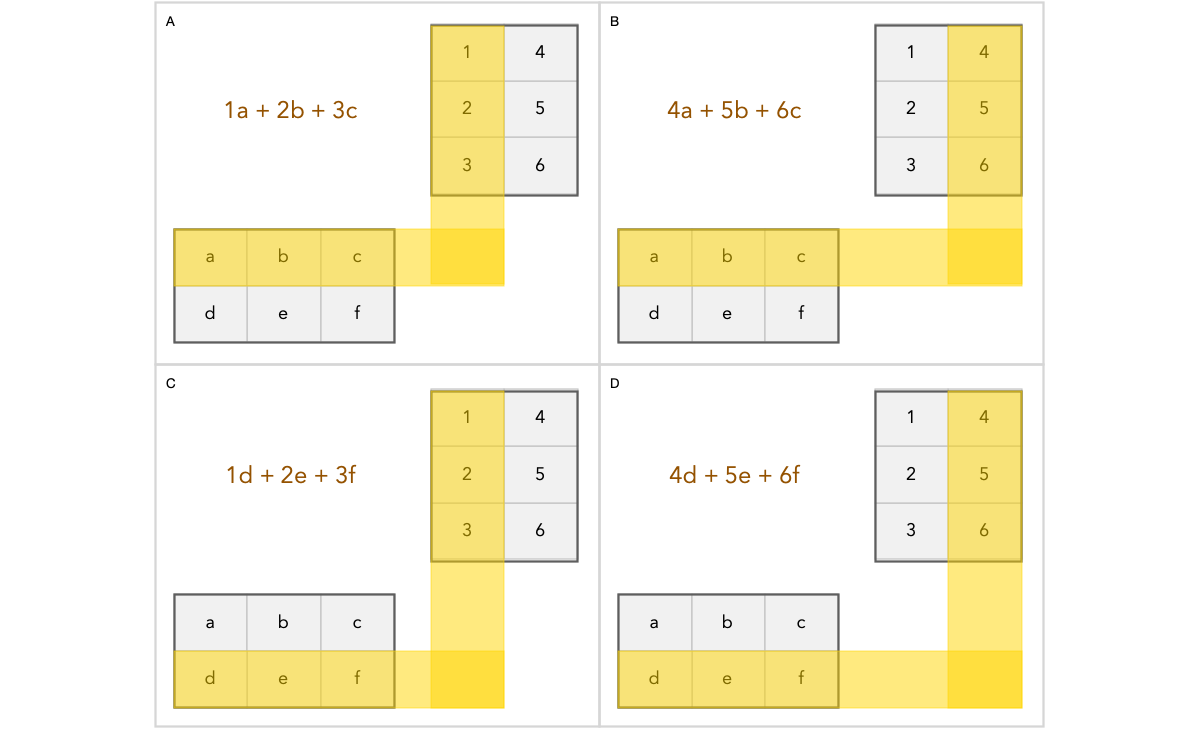# Why Does Matrix Multiplication Work the Way it Does?Multiplying a row vector with a column vectorDemonstrate how each cell is calculated in the result matrix when multiplying a column vector with a row vector.Shows which rows and columns will be combined to calculate a specific cell in the result matrix. In brown color you see the calculated result which will be stored in that cell.
`v₁, v₂, ..., vₙ`
`c₁, c₂, ..., cₙ`
`y = c₁v₁ + c₂v₂ + ..., cₙvₙ`
`[a, x], [b, y], [c, z]`
`2, 4, 6`

--

--